### Statistical Soup: ANOVA, ANCOVA, MANOVA, & MANCOVA

August 11, 2014 Jeremy J. Taylor

Copied verbatim from here.

The distinctions between ANOVA, ANCOVA, MANOVA, and MANCOVA can be difficult to keep straight. Before one can appreciate the differences, it is helpful to review the similarities among them.

ANOVA

The core component of all four of these analyses (ANOVA, ANCOVA, MANOVA, AND MANCOVA) is the first in the list, the ANOVA. An “Analysis of Variance” (ANOVA) tests three or more groups for mean differences based on a continuous (i.e. scale or interval) response variable (a.k.a. dependent variable). The term “factor” refers to the variable that distinguishes this group membership. Race, level of education, and treatment condition are examples of factors.

There are two main types of ANOVA: (1) “one-way” ANOVA compares levels (i.e. groups) of a single factor based on single continuous response variable (e.g. comparing test score by ‘level of education’) and (2) a “two-way” ANOVA compares levels of two or more factors for mean differences on a single continuous response variable(e.g. comparing test score by both ‘level of education’ and ‘zodiac sign’). In practice, you will see one-way ANOVAs more often and when the term ANOVA is generically used, it often refers to a one-way ANOVA. Henceforth in this blog entry, I use the term ANOVA to refer to the one-way flavor.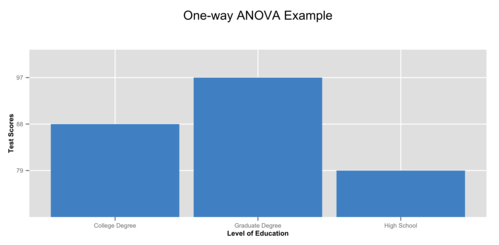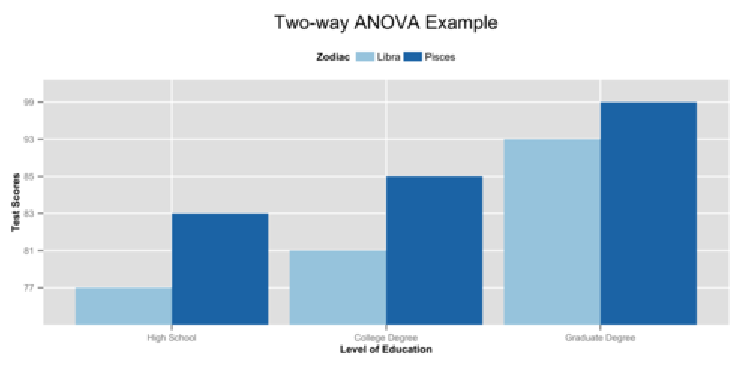ANCOVA

The obvious difference between ANOVA and ANCOVA is the the letter “C”, which stands for ‘covariance’. Like ANOVA, “Analysis of Covariance” (ANCOVA) has a single continuous response variable. Unlike ANOVA, ANCOVA compares a response variable by both a factor and a continuous independent variable (e.g. comparing test score by both ‘level of education’ and ‘number of hours spent studying’). The term for the continuous independent variable (IV) used in ANCOVA is “covariate”.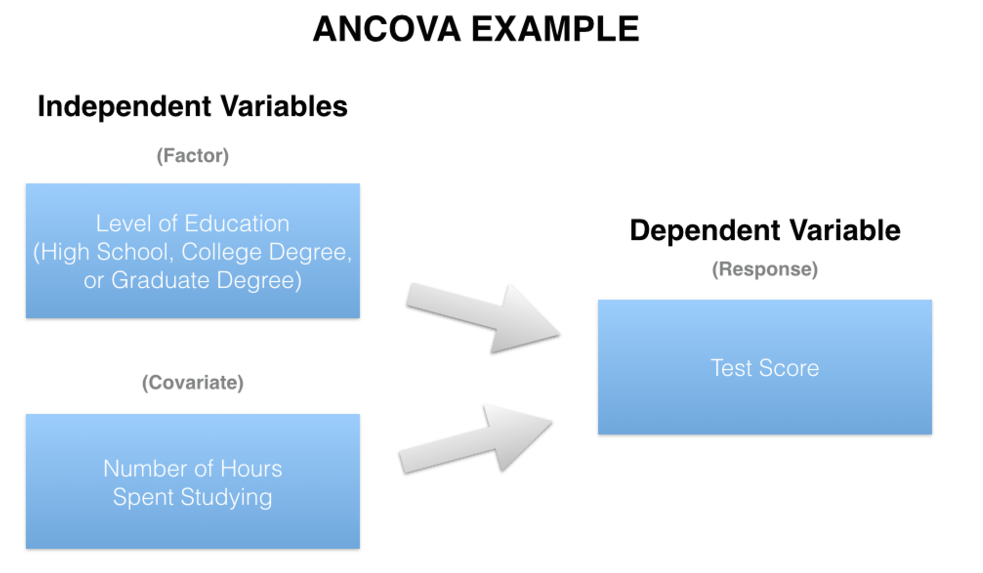ANCOVA is also commonly used to describe analyses with a single response variable, continuous IVs, and no factors. Such an analysis is also known as a regression. In fact, you can get almost identical results in SPSS by conducting this analysis using either the “Analyze > Regression > Linear” dialog menus or the “Analze > General Linear Model (GLM) > Univariate” dialog menus.

A key (but not only) difference in these methods is that you get slightly different output tables. Also, regression requires that user dummy code factors, while GLM handles dummy coding through the “contrasts” option. The linear regression command in SPSS also allows for variable entry in hierarchical blocks (i.e. stages).

MANOVA

The obvious difference between ANOVA and a “Multivariate Analysis of Variance” (MANOVA) is the “M”, which stands for multivariate. In basic terms, A MANOVA is an ANOVA with two or more continuous response variables. Like ANOVA, MANOVA has both a one-way flavor and a two-way flavor. The number of factor variables involved distinguish a one-way MANOVA from a two-way MANOVA.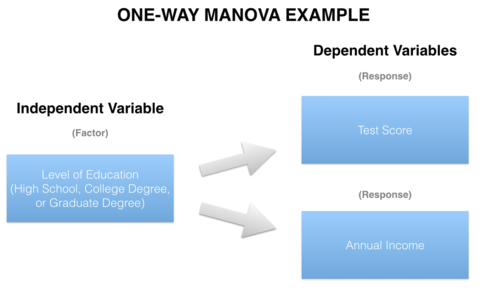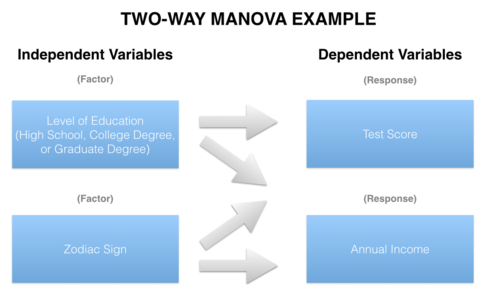When comparing two or more continuous response variables by a single factor, a one-way MANOVA is appropriate (e.g. comparing ‘test score’ and ‘annual income’ together by ‘level of education’). A two-way MANOVA also entails two or more continuous response variables, but compares them by at least two factors (e.g. comparing ‘test score’ and ‘annual income’ together by both ‘level of education’ and ‘zodiac sign’).

A more subtle way that MANOVA differs from ANOVA is that MANOVA compares levels of a factor that has only two levels (a.k.a. binary). When dealing with a single response variable and binary factor (e.g. gender), one uses an independent sample t-test. However, a t-test can not estimate differences for more than one response variable together, thus a MANOVA fills that need.

MANCOVA

Like ANOVA and ANCOVA, the main difference between MANOVA and MANCOVA is the “C,” which again stands for “covariance.” Both a MANOVA and MANCOVA feature two or more response variables, but the key difference between the two is the nature of the IVs. While a MANOVA can include only factors, an analysis evolves from MANOVA to MANCOVA when one or more more covariates are added to the mix.